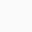# Find the Smallest 6 digit number which is exactly divisible by 175

The question is reprouduced here.

Find the smallest 6 digit number Which is exactly divisible by 175

 smallest 6-digit number= 100000 Hint: smallest 6-digit number is 1 with 0 written 5 times. Smallest 1-digit number is 1. Smallest 2-digit number is 10 , Smallest 3-digit number is 100 and so on . 100000/175 Quotient= 571 Remainder= 75 100 more can divide it exactly and will remain smallest 6 digit number. Therefore, The required number = 100000+100 =100100 Hint: Remainder is the amount in the dividend (100000). Therefore this excess amount when added to 100 to get the exact dividend divisible by 175.

##### 2 thoughts on “Find the Smallest 6 digit number which is exactly divisible by 175”
1.Azra Ahmad says:

Thanks for reminding, but the sum has been corrected. The number will be 100100 . This will be exactly divided with quotient 572.

2.allfree4u says:

Dear Sir,
As you have mentioned already, the smallest 6 digit number is 100000, so any number subtracted from that will be " more small" and become a 5 digit number.
So, we have to add any number greater that smallest number to get the number divisible by 175 exactly.
Therefore, 571-75=496 is the number we have to add with 100000 to have 100496 and this is the required number as par the question.
Thanks,
Subhangi Roy, Techno India Gp.Public School , Hooghly, W.B